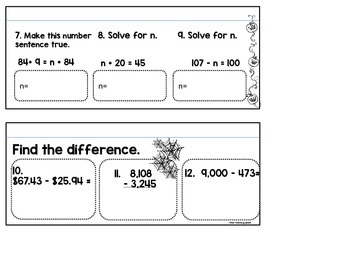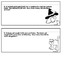Subject
Resource Type
File Type
PDF (1 MB)
\$3.00
Product Description
Get your kiddos “Going Batty” over math with this fun Halloween themed booklet.

Students are tasked with completing addition and subtraction problems and then coloring and cutting out the booklet to create a bat. To assemble, simply staple the pages behind the bat.

There are two different book choices included for your differentiation needs..

Set A Skills- 16 Problems with Answer Key
3 Problems: Create an example of the commutative, associative, and identity properties of addition given a set of numbers.
3 Problems: Solve for the missing digit with one and two digit numbers.
3 Problems: Find the sum of three digit numbers with regrouping.
3 Problems: Find the difference of three digit numbers with regrouping and subtraction over zeros.
4 word problems that require students to decided whether to add or subtract three digit numbers.

TO PRINT SET A: choose pages 3-7 Answer Key: pages 8-12

Set B Skills- 16 Problems with Answer Key
3 Problems: Create an example of the commutative, associative, and identity properties of addition given a set of numbers.
3 Problems: Solve for the missing digit with two and three digit numbers.
3 Problems: Find the sum of four digit numbers with regrouping.
3 Problems: Find the difference of four digit numbers with regrouping and subtraction over zeros.
4 word problems that require students to decided whether to add or subtract four digit numbers.

TO PRINT SET B: choose pages 13-17 Answer Key: 18-22

*****************************************************************************
If you love this differentiated theme book? Then check out my differentiated Haunted Restaurant Project here

Total Pages
N/A